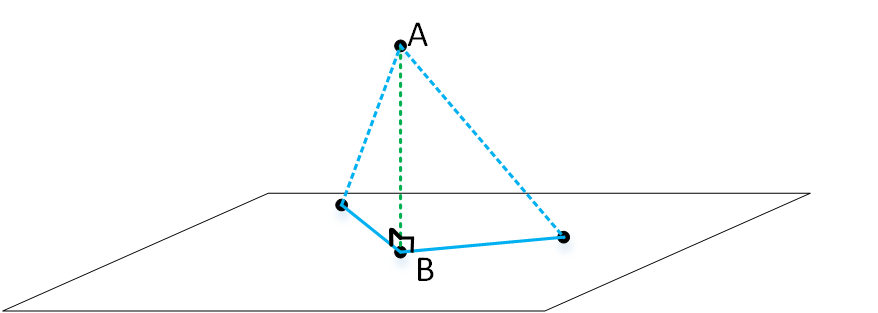# 模型估计



$$\left\{ \begin{eqnarray} \hat{b} &=& \frac{\sum_{i=1}^n(x_i-\bar{x})(y_I-\bar{y})}{\sum_{i=1}^n(x_i-\bar{x}^2)} = \frac{\sum_{i=1}^nx_iy_i-n\bar{x}\bar{y}}{\sum_{i=1}^nx_i^2-n\bar{x}^2} \\ \hat{a} &=& \bar{y}-\hat{b}\bar{x} \\ \end{eqnarray} \right.$$

$$\mathbf{A}\mathbf{x}=\mathbf{b}$$

$$\arg\min_{\mathbf{x}} \|\mathbf{A}\mathbf{x}\|_2$$或$$\arg\min_{\mathbf{x}} \|\mathbf{A}\mathbf{x}-\mathbf{b}\|_2$$

### SVD分解

$$\mathbf{A} = \mathbf{U}\mathbf{D}\mathbf{V}^T$$
$$\mathbf{U}$$和$$\mathbf{V}$$都是正交矩阵（严格来说，是酉矩阵），$$\mathbf{D}$$为对角矩阵，而且对角线上的元素非负，从左上角到右下角递减。至于SVD为什么存在，移步维基百科。$$\mathbf{V}$$的最后一列就是使得$$\|\mathbf{A}\mathbf{x}\|_2$$取得最小值的单位向量。利用SVD的表达式，要验证这一点并不难。因为$$\mathbf{V}$$是正交矩阵，所以它的所有列$$\{\mathbf{v}_k\}$$构成$$\mathbf{x}$$所在空间的一组标准正交基，于是$$\mathbf{x} = \sum_{k}a_k\mathbf{v}_k$$。设$$\mathbf{D}=\mathrm{diag}\{\lambda_k\}$$，利用SVD表达式计算$$\mathbf{Ax}$$的范数的平方
$$\begin{eqnarray} \|\mathbf{Ax}\|_2^2 &=& \|\mathbf{UDV}^T\sum_ka_k\mathbf{v}_k\|_2^2 \\ &=& \|\mathbf{DV}^T\sum_ka_k\mathbf{v}_k\|_2^2 \quad\quad （\mathbf{U}\text{具有保范性}）\\ &=& \|\mathbf{D}\sum_ka_k\mathbf{V}^T\mathbf{v}_k\|_2^2 \quad\quad （\text{利用}\mathbf{V}的正交性）\\ &=& \sum_k|\lambda_ka_k|_2^2 \quad\quad （\text{利用}\mathbf{V}的正交性和\mathbf{D}的对角性）\\ &\geq& \sum_k|\lambda_na_k|_2^2 \quad\quad （\lambda_{k+1}\geq\lambda_k）\\ &=& \lambda_n^2 \quad\quad （\mathbf{x}为单位向量） \end{eqnarray}$$

### 最小二乘### 参数估计举例

x = [1:5];
y = 3*x + 4 + randn(1,5);


 $$x$$ 1 2 3 4 5 $$y$$ 6.795 9.87592 14.4897 17.409 20.4172

n = length(x);
A = zeros(n,3);
for i=1:length(x)
A(i,:) = [x(i) 1 -y(i)];
end
[~,~,v] = svd(A);
v_end = v(:,end);
v_end = v_end/v_end(3);
k = v_end(1)
d = v_end(2)


n = length(x);
A = zeros(n,2);
b = zeros(n,1);
for i=1:length(x)
A(i,:) = [x(i) 1];
b(i,:) = [y(i)];
end
result = (A'*A)\A'*b;
k = result(1)
d = result(2)


 $$k$$ $$d$$ SVD 3.4551 3.4549 最小二乘 3.4777 3.3641